﻿ Exoplanets — Keep Talking and Nobody Explodes Module## On the Subject of Exoplanets

Balenci’, Balenci’, Balenci’, Balenci’, Balenci’, Balenci’, Balenci’, Balenci’, Balenci’, Balenci’, Balenci’, Balenci’, Balenci’, Balenci’, Balenci’.

• On the module is a star, and three planets orbiting it. To solve the module, press the “target planet” when the last digit of the countdown timer is the “target digit”.
• The starting target planet is the planet that’s orbiting in a different direction than the other planets. If all the planets are orbiting clockwise, the starting planet is the one closest to the star. If all the planets are orbiting counterclockwise, the starting planet is the one farthest from the star. The starting target digit is the surface value of the target planet, as determined by the planet’s appearance.
 0 1 2 3 4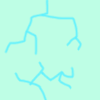5 6 7 8 9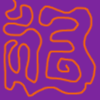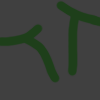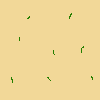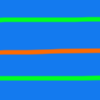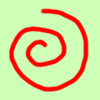• Now, modify the target planet and target digit using the table on the next page. From the cell labelled “start”, start by facing north, then turn 45° in the direction the star is spinning for every battery on the bomb. Move in that direction to the cell in the ring of the starting target planet (for example, the outermost planet corresponds to the outermost ring in the table).
• Move, in the direction the planet is orbiting, the orbital period of the planet plus its surface value modulo the number of battery holders, then add the number of ports. If there are no battery holders, modulo by 5. Apply the rule that corresponds to the letter that was landed on.
• Move to the next ring/planet inward (if the current ring is the inner ring, move to the outer ring) and continue performing the above step until a modification has been carried out for all three planets.

Note: The orbital period of an object is the amount of time it takes to complete a full revolution around an object, which in this case is the star. The possible orbital periods in this module are 5, 10, 20, and 40, all in seconds.

Letter: Modification:
A Target planet is the smallest planet that’s larger than the current target planet. If the current target planet is the largest planet, the target planet is the smallest planet.*
B Target digit is the current target digit plus the starting target digit, mod 10.
C Target planet is the largest planet that’s smaller than the current target planet. If the current target planet is the smallest planet, the target planet is the largest planet.*
D Target planet is the planet whose value is furthest from the current target planet’s value.*
E Target digit is nine minus the current target digit.
F Target planet is the planet closest to the star that is orbiting in the opposite direction of the current target planet.*
G Target planet is the planet closest to the star that is orbiting in the same direction as the current target planet.*
H Target digit is the current target digit plus the sum of all the serial number digits, mod 10.
I Target planet is the planet with the smallest value that is still greater than the target planet’s value. If the current target planet has the greatest value, the target planet is the planet with the smallest value*.
J Target digit is the current target digit plus the number of modules on the bomb, mod 10.
K Target planet is the planet one ring inward from the current target planet. If the current target planet is the innermost planet, the target planet is the outermost planet.*
L Target digit is the current target digit plus the first serial number digit, mod 10.
M Target planet is the planet whose value is closest to the current target planet’s value.*
Letter: Modification:
N Target digit is the current target digit plus the second serial number digit, mod 10.
O Target planet is the slowest-orbiting planet that orbits faster than the current target planet. If the current target planet is the fastest-orbiting planet, the target planet is the slowest-orbiting planet.*
P Target planet is the planet furthest from the current target planet.*
Q Target digit is the current target digit plus the number of ports, mod 10.
R Target digit is the current target digit plus five, mod 10.
S Target planet is the fastest-orbiting planet that orbits slower than the current target planet. If the current target planet is the slowest-orbiting planet, the target planet is the fastest-orbiting planet.*
T Target digit is the current target digit plus the last serial number digit, mod 10.
U Target planet is the planet one ring outward from the current target planet. If the current target planet is the outermost planet, the target planet is the innermost planet.*
V Target digit is the current target digit plus the number of batteries, mod 10.
W Target digit is the current target digit plus the number of consonants in indicators, mod 10.
X Target planet is the planet with the greatest value that is still smaller than the target planet’s value. If the current target planet has the smallest value, the target planet is the planet with the greatest value*.

* If there are multiple planets that fit the criterion, use the planet out of those that is closest to the star. If there are no planets that fit the criterion, use the inner planet.